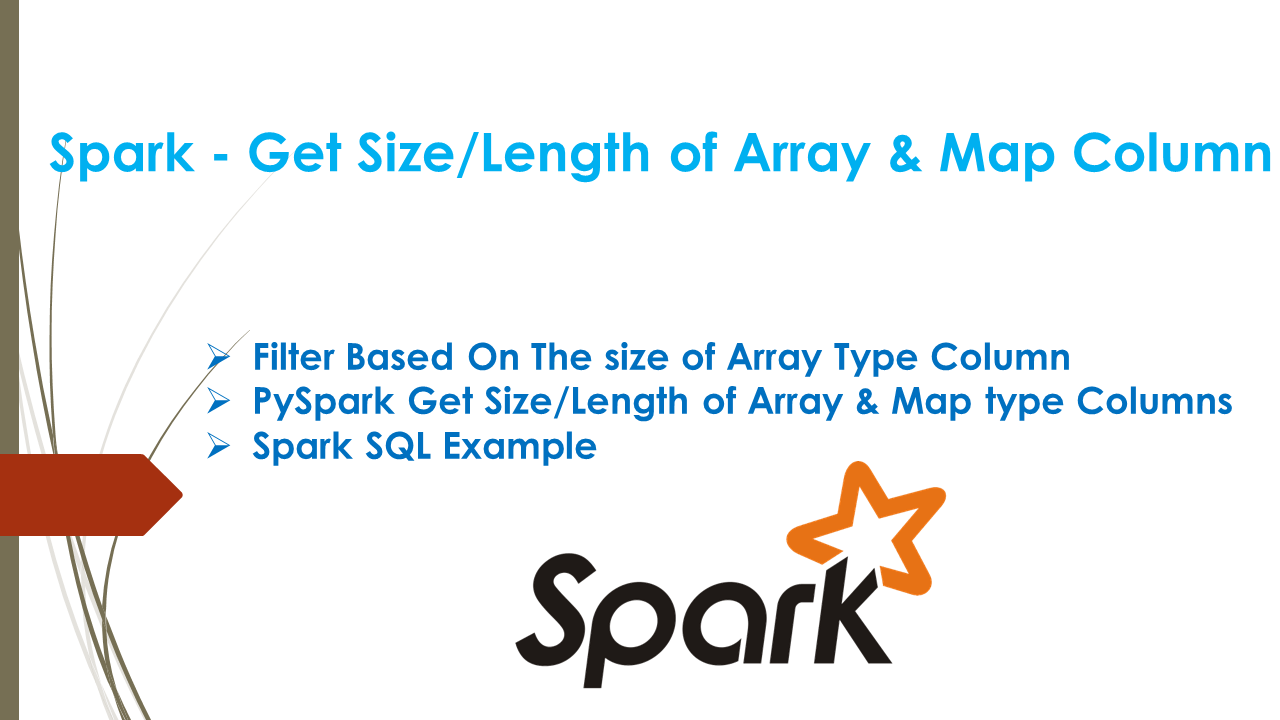# Spark – Get Size/Length of Array & Map Column

Question: In Spark & PySpark, how to get the size/length of ArrayType (array) column and also how to find the size of MapType (map/Dic) type in DataFrame, could you also please explain with an example how to filter by array/map size?

PySpark Example: How to Get Size of ArrayType, MapType Columns in PySpark

## 1. Solution: Get Size/Length of Array & Map DataFrame Column

Spark/PySpark provides `size()` SQL function to get the size of the array & map type columns in DataFrame (number of elements in ArrayType or MapType columns). In order to use Spark with Scala, you need to import `org.apache.spark.sql.functions.size` and for PySpark `from pyspark.sql.functions import size`, Below are quick snippet’s how to use the size() function.

Note: By default this function return -1 for null array/map columns. To change this behavior and if you want to get null for null input set false to `spark.sql.legacy.sizeOfNull` or true to `spark.sql.ansi.enabled`.

``````
// Filter based on array size of a column
import org.apache.spark.sql.functions.{size,col}
df.filter(size(col("languages")) > 1).show()

// Size of ArrayType & MapType columns
df.withColumn("lang_len",size(col("languages")))
.withColumn("prop_len",size(col("properties")))
.show()

// Using size() on Spark SQL.
df.createOrReplaceTempView("TAB")
spark.sql("select name,size(languages) as lang_len from TAB" +
" where size(languages) > 1").show()
``````

Let’s see with a detailed example

``````
val arrayData = Seq(
Row("James",List("Java","Scala"),Map("hair"->"black","eye"->"brown")),
Row("Michael",List("Spark","Java",null),Map("hair"->"brown","eye"->null)),
Row("Robert",List("CSharp"),Map("hair"->"red")),
Row("Washington",null,null),
Row("Jeferson",List(),Map())
)

val arraySchema = new StructType()

val df = spark.createDataFrame(
spark.sparkContext.parallelize(arrayData),arraySchema)
``````

## 2. Filter Based On The size of Array Type Column

On the above Spark DataFrame, we have a column `languages` of type Array & `properties` of type Map, below example shows how to filter DataFrame that has a number of elements in `languages` column > 1.

``````
// Filter Dataframe using size() of a column
import org.apache.spark.sql.functions.{size,col}
df.filter(size(col("languages")) > 1).show(false)

// +-------+--------------+-----------------------------+
// |name   |languages     |properties                   |
// +-------+--------------+-----------------------------+
// |James  |[Java, Scala] |[hair -> black, eye -> brown]|
// |Michael|[Spark, Java,]|[hair -> brown, eye ->]      |
// +-------+--------------+-----------------------------+
``````

Another example that creates a new column with the size of array and map columns. note that when the column values are null it returns size as -1.

``````
// Get the size of a column to create anotehr column
df.withColumn("lang_len",size(col("languages")))
.withColumn("prop_len",size(col("properties")))
.show(false)

// +----------+--------------+-----------------------------+--------+--------+
// |name      |languages     |properties                   |lang_len|prop_len|
// +----------+--------------+-----------------------------+--------+--------+
// |James     |[Java, Scala] |[hair -> black, eye -> brown]|2       |2       |
// |Michael   |[Spark, Java,]|[hair -> brown, eye ->]      |3       |2       |
// |Robert    |[CSharp]      |[hair -> red]                |1       |1       |
// |Washington|null          |null                         |-1      |-1      |
// |Jeferson  |[]            |[]                           |0       |0       |
// +----------+--------------+-----------------------------+--------+--------+
``````

## 3. PySpark Get Size/Length of Array & Map type Columns

In PySpark size() function is available by importing `from pyspark.sql.functions import size` get the number of elements in a Array or Map type columns.

``````
# Filter Dataframe using size() of a column
from pyspark.sql.functions import size,col
df.filter(size("languages") > 2).show(truncate=False)

# Get the size of a column to create another column
df.withColumn("lang_len",size(col("languages")))
.withColumn("prop_len",size(col("properties")))
.show(false)
``````

## 4. Spark SQL Example

If you are using Spark SQL, you can also use `size()` function that returns the size of an array or map type columns. The Below example creates a new column `lang_len` with the size of the array column `language` and filters the DataFrame that has minimum languages 2.

``````
// Using size() function on SQL
df.createOrReplaceTempView("TAB")
spark.sql("select name,size(languages) as lang_len from TAB" +
" where size(languages) > 1").show(false)

// +-------+--------+
// |name   |lang_len|
// +-------+--------+
// |James  |2       |
// |Michael|3       |
// +-------+--------+
``````

## 5. Conclusion

In summary SQL function size() is used to get the number of elements in array or map type DataFrame columns and this function return by default -1 for null values and you can change this behavior by setting spark config.

Happy Learning !!

### Naveen (NNK)

Naveen (NNK) is a Data Engineer with 20+ years of experience in transforming data into actionable insights. Over the years, He has honed his expertise in designing, implementing, and maintaining data pipelines with frameworks like Apache Spark, PySpark, Pandas, R, Hive and Machine Learning. Naveen journey in the field of data engineering has been a continuous learning, innovation, and a strong commitment to data integrity. In this blog, he shares his experiences with the data as he come across. Follow Naveen @ @ LinkedIn